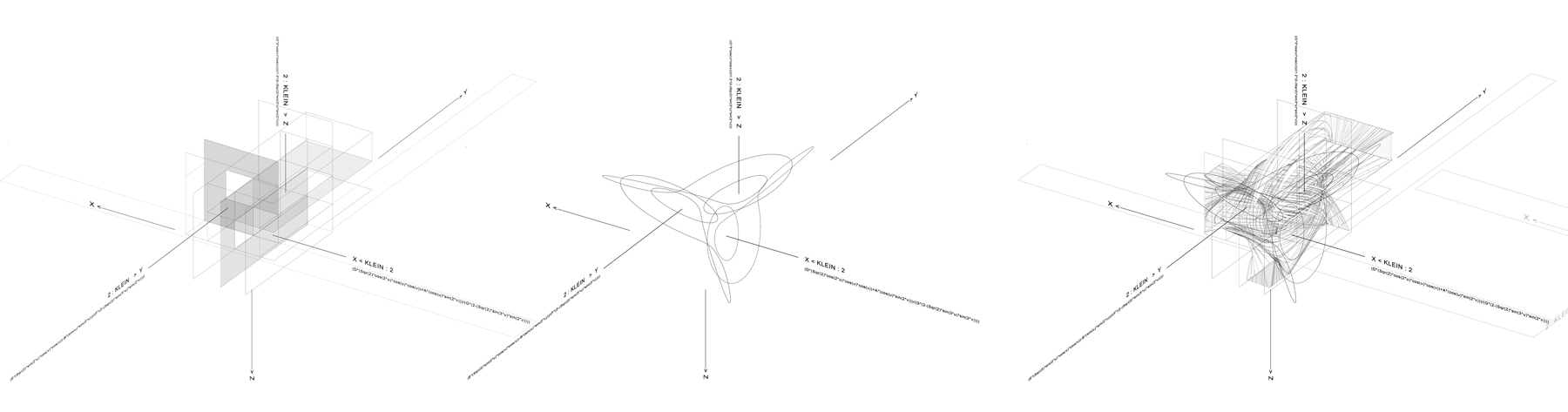# Pablo Lorenzo-Eiroa

Associate Professor Adj., Design Principal e-Architects
The School of Architecture The Cooper Union
New York City, NY 10003, USA

Multidimensional Space
Spatial organization plays an essential role at a cognitive level, presenting limits to how architects and other disciplines measure and reference dimensions in space. House IIB is an experimental project that integrates two topological mathematical surfaces. Each of the three Cartesian planes (XY, ZY, ZX) propose an initial organization with a central void. Each of the centers and corners of the planes become continuous with each other following a Boy Topology. A Boy Surface can be mathematically scripted through various degrees of curvature. This experimental house integrates two forms of scripting the surface, as straight lines and as curves, discovering that they can become continuous with each other.Multidimensional Space: -X, X -Y, Y -Z, Z
40 x 40 cm
Wolfram Mathematica, Mathematical Script Rhino, Autodesk Maya
2013-2016

Multidimensional Space: -X, X -Y, Y -Z, Z
House II version B is an architecture experimental house project that integrates within a single mathematical surface a twelve-fold-surface topology, interconnecting two mathematical Boy Surfaces. A first mathematical surface integrates three Klein topologies and six Möbius topologies into a continuous sequence. The surface is scripted to reference but also displace the three Cartesian coordinate planes XY-YZ-XZ. The first mathematical surface is developed through double curvature, the second mathematical surface contains the first one and is developed as a series of three Cartesian continuous volumes where interior and exterior, center and periphery are continuous with each other.